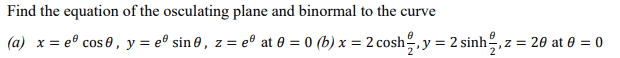# Vector calculus

• MHB
WMDhamnekar
MHBI am working on this question. Any math help, hint or even correct answer will be accepted.

WMDhamnekar
MHB
Hi,
Answer to question (a): The equation of the osculating plane at $\theta=0$ is $-\sqrt{\frac16}x + \sqrt{\frac23}z -\sqrt{\frac16}=0$ and binormal $\hat{B}(\theta=0)=\left[-\sqrt{\frac16},-\sqrt{\frac16},\sqrt{\frac23}\right]$

Is this answer correct? In my opinion, it should be correct.

Answer (b):$\vec{r}(\theta)=\left[2\cosh{\frac{\theta}{2}}, 2\sinh{\frac{\theta}{2}},2\theta\right] \implies \vec{r'}(\theta)=\left[\sinh{\frac{\theta}{2}}, \cosh{\frac{\theta}{2}},2\right],||\vec{r'}(\theta)||=\sqrt{\sinh^2{\frac{\theta}{2}}+\cosh^2{\frac{\theta}{2}}+4}$
$||\vec{r'}(\theta)||_{(\theta=0)}=\sqrt{[0,1,4]}=\sqrt{5}, \hat{T}(\theta)=\left[\frac{\sinh{\frac{\theta}{2}}}{\sqrt{\sinh^2{\frac{\theta}{2}}+\cosh^2{\frac{\theta}{2}}+4}}, \frac{\cosh{\frac{\theta}{2}}}{\sqrt{\sinh^2{\frac{\theta}{2}}+\cosh^2{\frac{\theta}{2}}+4}},\frac{2}{ \sqrt{\sinh^2{\frac{\theta}{2}}+\cosh^2{\frac{\theta}{2}}+4}} \right],\hat{T}(\theta)_{(\theta=0)}=[0,\frac{1}{\sqrt{5}},\frac{2}{\sqrt{5}}]$

Equation of the osculating plane at $\theta=0$ is 0 and binormal to the curve at point (2,0,0) is $\left[0,\frac{2}{\sqrt{5}},-\frac{1}{\sqrt{5}}\right]$

Last edited: